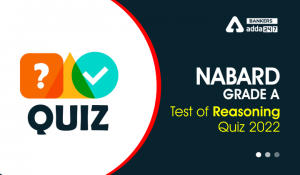Latest Banking jobs   »   Reasoning Ability Quiz For SBI Clerk...

# Reasoning Ability Quiz For SBI Clerk Prelims 2021- 26th May

(1-5): Study the following arrangement carefully and answer the questions given below:
N 4 7 T U J K 3 % F @ © L N 5 P 1 8 U \$ E 2 D C 6 # 9 Z Q 8 Y*M A

Q1. Which of the following element is sixth to the left of the fifteenth from the left end of the given arrangement?
(a) 2
(b) #
(c) %
(d) \$
(e) None of these

Q2. How many such numbers are there in the given arrangement each of which is immediately preceded by a consonant but not immediately followed by a letter?
(a) One
(b) None
(c) Three
(d) Two
(e) Four

Q3. How many such symbols are there in the arrangement, each of which is immediately followed by a letter but not immediately preceded by a number?
(a) One
(b) Three
(c) None
(d) Two
(e) More than three

Q4. If we remove all the numbers from the given arrangement, then which of the following element is 5th from the right end?
(a) Q
(b) T
(c) *
(d) #
(e) None of these

Q5. Four of the following five are alike in a certain way based on their positions in the given arrangement and so from a group. Which is the one that does not belong to that group?
(a) DC6
(c) 9ZQ
(d) 5P1
(e) #MP

Directions (6-10): Study the following arrangement carefully and answer the questions given below:
3 1 2 5 4 7 2 8 4 5 7 1 9 6 5 2 4 1 4 5 8 2 4 3 9 7 6 9 4 2 3

Q6. Which of the following digit will be sixth to the left of tenth from the right end?
(a) 6
(b) 9
(c) 5
(d) 2
(e) None of these

Q7. How many 2’s is there in the given series which are followed by a number less than 6?
(a) One
(b) None
(c) Three
(d) Two
(e) More than three

Q8. What will be the addition of fifth, seventh and tenth digit from the left end?
(a) 13
(b) 10
(c) 11
(d) 15
(e) None of these

Q9. If all the perfect square digits are removed from the given series, then which of the following will be fifth to the right of sixth from the left end?
(a) 5
(b) 2
(c) 8
(d) 1
(e) None of these

Q10. How many odd numbers are there in the given series which are immediately followed by a perfect square digit?
(a) One
(b) None
(c) Three
(d) Five
(e) Seven

Directions (11-15): These questions are based on the following alphabet and digit series.
D 5 P K L 8 Z M C I 6 O Q U E 1 T N R 4 G B A 9 F S W 2 H J V 7 Y X 3

Q11. How many digits are there in above arrangement which is immediately followed by vowel?
(a) Two
(b) Four
(c) One
(d) Three
(e) None

Q12. If all the vowels are deleted from the above arrangement, then which of the following letter/digit is 10th to left of 5th from the right end?
(a) R
(b) 4
(c) G
(d) 9
(e) None of these

Q13. Which of the following letter/digit is 7th to the right of 13th from the right end?
(a) A
(b) 2
(c) H
(d) 9
(e) None of these

Q14. How many consonants are there in above arrangement which is immediately preceded by consonant and immediately followed by vowel?
(a) Four
(b) Three
(c) Two
(d) One
(e) None

Q15. Which of the following element is exactly between ‘B’ and ‘2’ in the given arrangement?
(a) 9
(b) F
(c) S
(d) W
(e) None of these

Practice More Questions of Reasoning for Competitive Exams:

###### SBI Clerk Study Plan 2021

Solutions

S1. Ans.(c)

S2. Ans.(e)
Sol. N47, K3%, C6#, P18

S3. Ans.(b)

S4. Ans.(a)

S5. Ans.(e)

Solutions (6-10):
S6. Ans(d)
Sol. The 16th element from the right end = ‘2’.

S7. Ans(e)
Sol. There are four 2’s which are followed by number less than 6 i.e. ‘2 5, 2 4, 2 4 and 2 3’.

S8. Ans(c)
Sol. The fifth, seventh and tenth digit from the left end are ‘4, 2 and 5′.
Hence, the sum is = (4+2+5)= 11.

S9. Ans (b)
Sol. After removing all the perfect square digits ‘3 2 5 7 2 8 5 7 6 5 2 5 8 2 3 7 6 2 3’
Then (6+5)= 11th digit from the left is ‘2’.

S10.Ans(e)
Sol. There are seven odd digits which are followed by a perfect square number- ‘3 1, 5 4, 7 1, 1 9, 1 4, 3 9, 9 4’’

Solutions (11-15):
S11. Ans(c)
Sol. ‘6 O’

S12. Ans(b)
Sol. D 5 P K L 8 Z M C 6 Q 1 T N R 4 G B 9 F S W 2 H J V 7 Y X 3
10th +5th = 15th from right = 4

S13. Ans(e)
Sol. 13th -7th = 6th from right = J

S14. Ans(c)
Sol. ‘M C I’, ‘G B A’

S15. Ans(b)#### Congratulations!Incorrect details? Fill the form again here

•Reasoning Ability Quiz For IBPS RRB PO C...
•Reasoning Ability Quiz For IBPS Clerk Pr...
•Reasoning Ability Quiz For NABARD Grade ...
•Reasoning Ability Quiz For IBPS RRB PO C...
•Reasoning Ability Quiz For IBPS Clerk Pr...
•Reasoning Ability Quiz For IBPS RRB PO C...